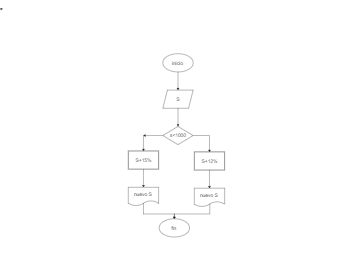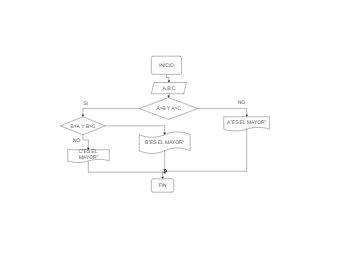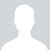## Example of Algorithm Flowchart

2
0
0
publish time: 2021-07-16Charlotte

The following examples of flowchart and algorithm shows the process to find the sums of the first 50 odd numbers. The sums of odd numbers from 1 to infinity can be calculated using Arithmetic Progression. The odd numbers are those numbers that are not divisible by 2. They are 1, 3, 5, 7, 9, 11, 14, 15, 17, 19, and so on. The sum of the first n odd numbers is Sn where Sn = 1+3+7+9+...+ (2n-1)...(1). As per AP, the sum of numbers is given by Sn = ½*n [2a+(n-1)d]...(2), where n = number of digits in the series, a = first term of an AP, and d = common difference in an Arithmetic Progression.

See More Related TemplatesHere is a math flowchart example. The flowchart is often used in representing algorithm, from which you could understand relative knowledge visually.
Math Flowchart Example
8
1
0Jose Luis Martinez Gonzaleztrabajo de diagrama de flujos
Dibujo1
17
0
0Sebastian Baron Lopez